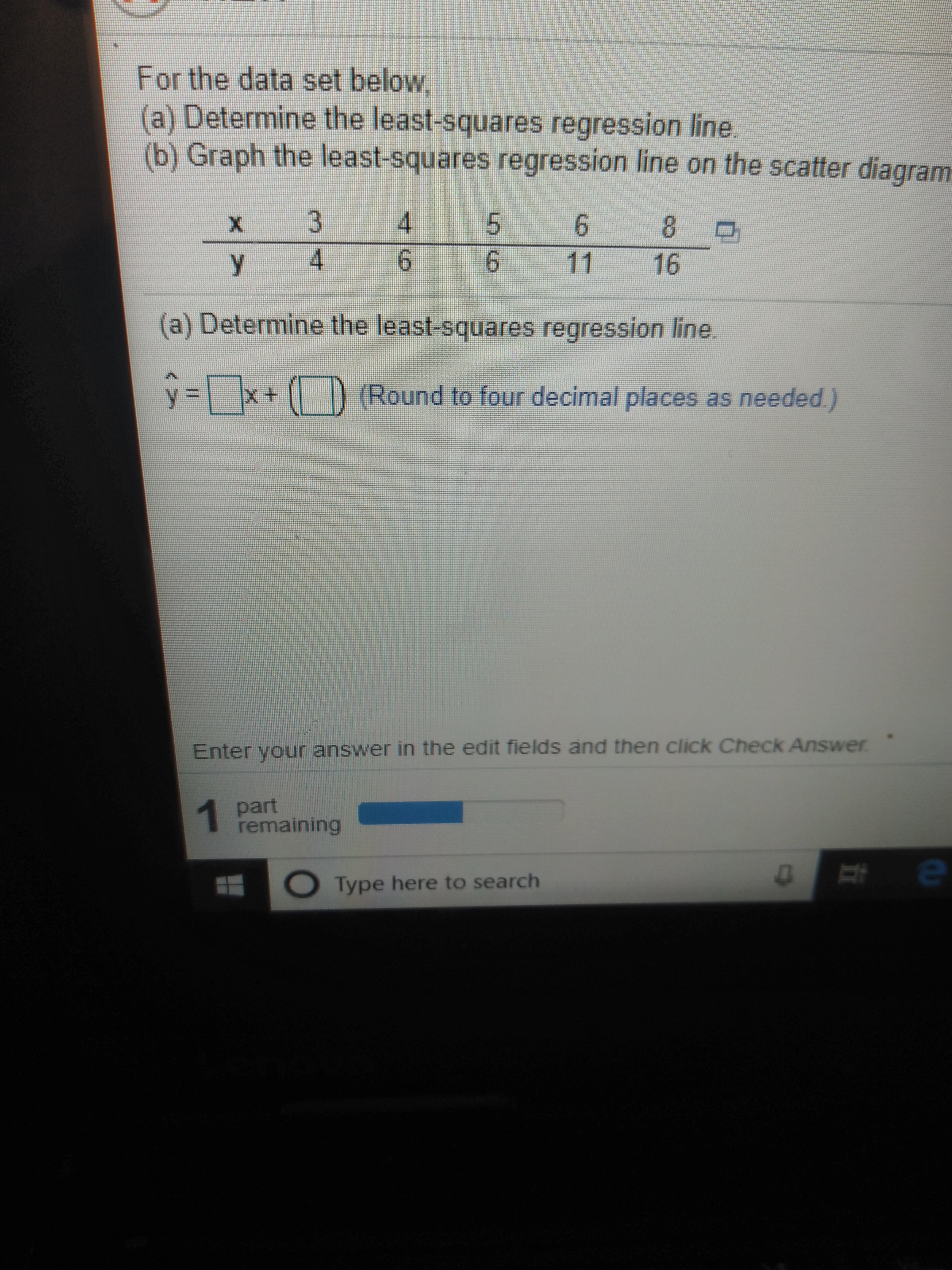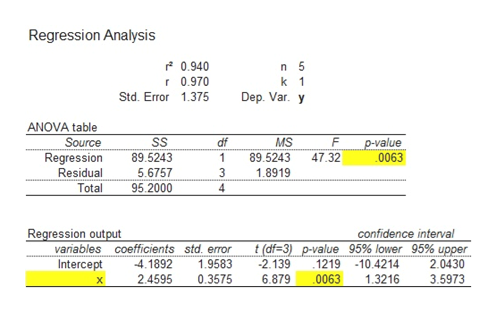# For the data set below(a) Determine the least-squares regression line(b) Graph the least-squares regression line on the scatter diagram3468461116(a) Determine the least-squares regression line) (Round to four decimal places as needed)y =xEnter your answer in the ed it fields and then click Check Answerpart1 remainingeType here to searchX

Question
49 viewshelp_outlineImage TranscriptioncloseFor the data set below (a) Determine the least-squares regression line (b) Graph the least-squares regression line on the scatter diagram 3 4 6 8 4 6 11 16 (a) Determine the least-squares regression line ) (Round to four decimal places as needed) y =x Enter your answer in the ed it fields and then click Check Answer part 1 remaining e Type here to search X fullscreen
check_circle

Step 1

a.

Step-by-step procedure to obtain the least square regression line using Excel Mega stat as follows:

• Choose MegaStat > Correlation/Regression > Regression Analysis.
• Select Input ranges, enter the values of X, Independent variable and enter the values of Y, Dependent variable.
• Check the appropriate options in Options.
• Click OK
Step 2

Output obtained using Excel Mega stat is represented as follows:help_outlineImage TranscriptioncloseRegression Analysis 0.940 n 5 r 0.970 k 1 Dep. Var. y Std. Error 1.375 ANOVA table Source Regression SS MS df P-value 0063 47.32 89.5243 89.5243 Residual 5.6757 1.8919 Total 95.2000 4 Regression output variables coefficients std. error confidence interval t(df-3) p-value 95% lower 95% upper 1219 0063 Intercept 4.1892 2.4595 1.9583 0.3575 2.139 6.879 10.4214 1.3216 2.0430 3.5973 fullscreen
Step 3

From the above output, the least square r...

### Want to see the full answer?

See Solution

#### Want to see this answer and more?

Solutions are written by subject experts who are available 24/7. Questions are typically answered within 1 hour.*

See Solution
*Response times may vary by subject and question.
Tagged in

### Statistics